# ISEE Upper Level Math : Prisms

## Example Questions

### Example Question #1 : Solve Problems Involving Area, Volume And Surface Area Of Two And Three Dimensional Objects: Ccss.Math.Content.7.G.B.6

Find the surface area of a non-cubic prism with the following measurements: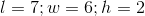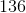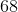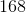Explanation:

The surface area of a non-cubic prism can be determined using the equation: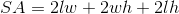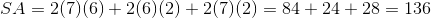### Example Question #1 : Prisms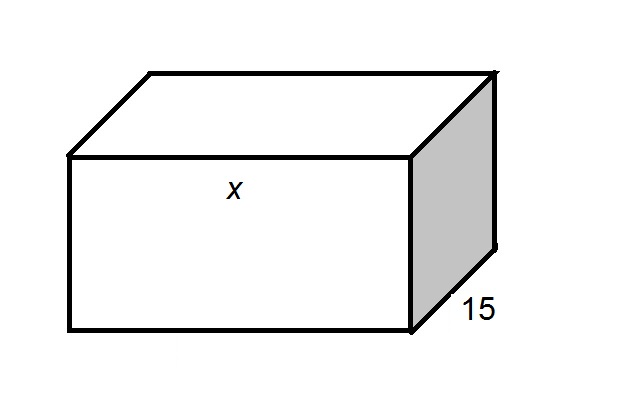The above diagram shows a rectangular solid. The shaded side is a square. In terms of, give the surface area of the box.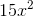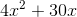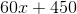Explanation:

A square has four sides of equal length, as seen in the diagram below.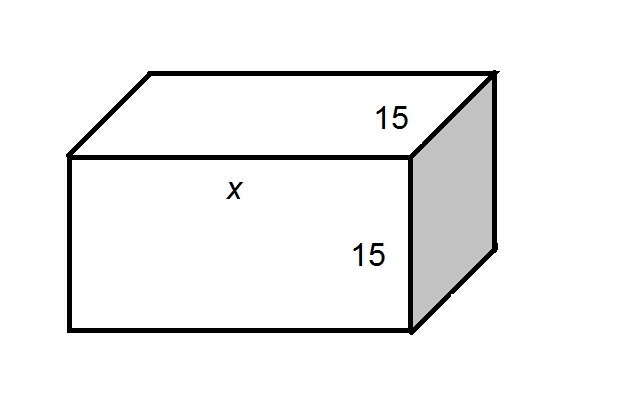All six sides are rectangles, so their areas are equal to the products of their dimensions:

Top, bottom, front, back (four surfaces):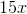Left, right (two surfaces):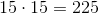The total surface area: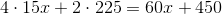### Example Question #21 : Solve Problems Involving Area, Volume And Surface Area Of Two And Three Dimensional Objects: Ccss.Math.Content.7.G.B.6

A rectangular prism has a width of 3 inches, a length of 6 inches, and a height triple its length. Find the volume of the prism.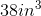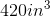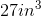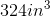Explanation:

A rectangular prism has a width of 3 inches, a length of 6 inches, and a height triple its length. Find the volume of the prism.

Find the volume of a rectangular prism via the following: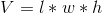Where l, w, and h are the length width and height, respectively.

We know our length and width, and we are told that our height is triple the length, so...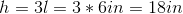Now that we have all our measurements, plug them in and solve: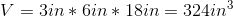### Example Question #2 : Solid GeometryThe above diagram shows a rectangular solid. The shaded side is a square. In terms of, give the volume of the box.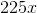Explanation:

A square has four sides of equal length, as seen in the diagram below.The volume of the solid is equal to the product of its length, width, and height, as follows: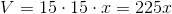.

### All ISEE Upper Level Math Resources